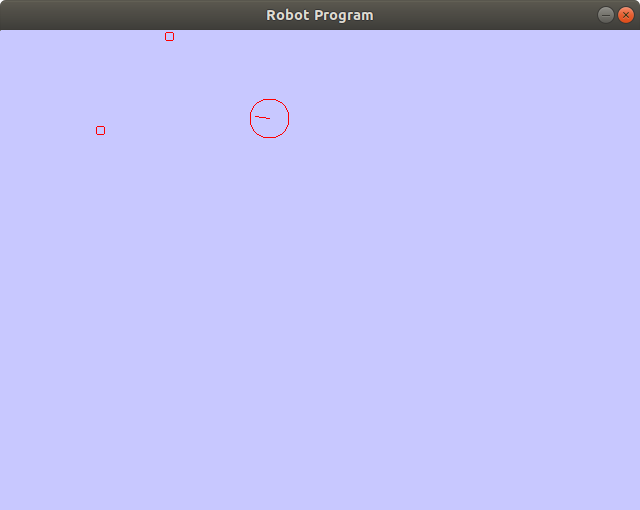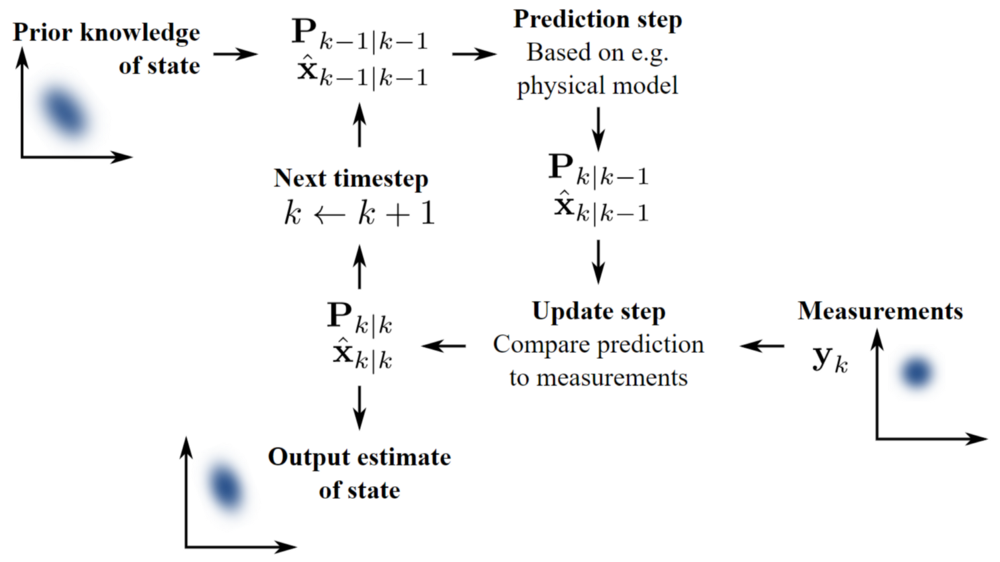Meet Robby. Robby is a robot. Well technically he is a simplistic virtual model of a robot, but that should be fine for our purpose. Robby is lost in his virtual world consisting of a two-dimensional plane and a couple of landmarks. He does have a map of his environment (no mapping is needed), but he does not know where exactly he is located in this environment.Robby (big red circle) and two landmarks (smaller red circles)

The purpose of this post is to walk you through the steps of robot localization using landmark detection and Extended Kalman Filtering.

# Part 1: Linear Kalman Filters

Kalman Filtering can be understood as a way of making sense of a noisy world. When we want to determine where a robot is located, we can rely on two things: We know how the robot moves from time to time since we command it to move in a certain way. This is called state transitioning (i.e. how the robot moves from one state to the other). And we can measure the robot’s environment using its various sensors such as cameras, lidar, or echolot. The problem is that both sets of information are subject of random noise. We do not know exactly how exactly the robot transitions from state to state since actuators are not perfect and we cannot measure the distance to objects with infinite precision. This is where Kalman Filtering comes to play.

Kalman Filtering allows us to combine the uncertainties regarding the current state of the robot (i.e. where it is located and in which direction it is looking) and the uncertainties regarding its sensor measurements and to ideally decrease the overall uncertainty of the robot. Both uncertainties are usually described by a Gaussian probability distribution, or Normal distribution. A Gaussian distribution has two parameters: mean and variance. The mean expresses, what value of the distribution has the highest probability to be true, and the variance expresses how uncertain we are regarding this mean value.

The algorithm works in a two-step process. In the prediction step, the Kalman filter produces estimates of the current state variables, along with their uncertainties. Once the outcome of the next measurement (necessarily corrupted with some amount of error, including random noise) is observed, these estimates are updated using a weighted average, with more weight being given to estimates with higher certainty. The algorithm is recursive. It can run in real time using only the present input measurements and the previously calculated state and its uncertainty matrix; no additional past information is required.

Since the Wikipedia image for the information flow in a Kalman Filter is so great, I cannot withheld it here:*Kalman Filtering. Image grabbed from the Kalman wiki page: *

2.png

I will not delve into the mathematical details of Kalman Filtering since many smart people already have done so. For a more in-depth explanation, I can recommend a stellar blog post by Tim Babb

# Part 2: Extended Kalman Filters

Extended Kalman Filtering is (as the name suggests) an extension of “Normal” Kalman Filtering. What I did not tell you in the last section is one additional assumption that was made implicitly wenn using Kalman Filters: The state transition model and the measurement model must be linear. From a mathematical standpoint this means that we can use the simplicity and elegance of Linear Algebra to update the robot’s state and the robot’s measurements. In practice, this means that the state variables and measured values are assumed to change linearly over time. For instance, if we measure the robot’s position in $$x$$-direction. We assume that if the robot was at position $$x_1$$ at time $$t_1$$, it must be at position $$x_1 + v (t_2–t_1)$$ at time $$t_2$$. The variable $$v$$ denotes the robot’s velocity in $$x$$-direction. If the robot is actually accelerating, or doing any other kind of nonlinear motion (e.g driving around in a circle), the state transition model is slighly wrong. Under most circumstances, it is not wrong by much, but in certain edge cases, the assumption of linearity is simply too wrong.

Also assuming a linear measurement model comes with problems. Assume you are driving along a straight road and there is a lighthouse right next to the road in front of you. While you are quite some distance away, your measurement of your distance to the lighthouse and the angle in which it lies from your perspective changes pretty much linearly (the distance decreases by roughly the speed your car has and the angle stays more or less the same). But the closer you get and especially while you drive past it, the angle, on one hand, changes dramatically, and the distance, on the other hand, does not change very much. This is why we cannot use Linear Kalman Filtering for Robby when he is navigating his 2-D world with landmarks scattered across his 2-D plane.

Extended Kalman Filter to the rescue! It removes the restriction of linear state transition and measurement models. Instead it allows you to use any kind of nonlinear function to model the state transition and the measurements you are making with your robot. In order to still be able to use the efficient and simple Linear Algebra magic in our filter, we do a trick: We linearize the models around the current robot state. This means that we assume the measurement model and the state transition model to be approximately linear around the state at which we are right now (refer to the road / lighhouse example again). But after every time step, we update this linearization around the new state estimate. While this approach forces us to make a linearization of this nonlinear function after every time step, it turns out to be not computationally expensive.

So there you have it. Extended Kalman Filtering is basically “Normal” Kalman Filtering just with additional linearization of the now nonlinear state transition model and measurement model.

In our case where Robby is lost and wants to localize in this (arguably) hostile environment, the Extended Kalman Filtering enables Robby to sense the landmarks and update its belief of its state accordingly. If the variance of the state estimate and the measurement estimate are low enough, Robby is very quickly very sure where he is located in respect to the landmarks and since he knows exactly where the landmarks are, he knows where he is!

His happiness-parameter is skyrocketing!

# Part 3: Implementation

The implementation in code is fairly straightforward. For visualization purposes, I chose the SDL2 Library for a quick-and-dirty visualization of all necessary objects. It can be downloaded here:

Following an object-oriented programming approach, I implemented the following classes:

• Class Robot

The Robot Class’ most important members are the Pose (x position, y position, and direction), and the Velocity (linear and angular velocity) . It can move forward, backward, and robtate left and right. For measuring the landmark positions, it has the method measureLandmarks, which takes the ground-truth landmarks, and overlays their position with fake measurement noise and returns a new list of measured landmarks.

class Robot {
public:
Robot(int x_start, int y_start, float orientation, int radius, SDL_Color col);
~Robot();

void render(SDL_Renderer * ren);
void move(const Uint8 * , Eigen::VectorXf & control);
void moveForward(Eigen::VectorXf & control);
void moveBackward(Eigen::VectorXf & control);
void rotateLeft(Eigen::VectorXf & control);
void rotateRight(Eigen::VectorXf & control);
void setPose(float x, float y, float phi);

Eigen::VectorXf get_state();
std::vector<Landmark> measureLandmarks(std::vector<Landmark> landmarks);

private:
Pose pose;
Velocity velocity;
SDL_Color color;
};

• Class KalmanFilter

The KalmanFilter class is arguably the most complex one. Its members are the matrices for state transitioning, measurements, and their respecive covariances. I will gloss over most of the details here, as the code comments give some hints about the purpose of most of the code. The filtering magic is happening in the localization_landmarks() member function.

class KalmanFilter {
public:

/**
* Create a Kalman filter with the specified matrices.
*   A - System dynamics matrix
*   C - Output matrix
*   Q - Process noise covariance
*   R - Measurement noise covariance
*   covariance - Estimate error covariance
*/

KalmanFilter(
double dt,
const Eigen::MatrixXf& A,
const Eigen::MatrixXf& C,
const Eigen::MatrixXf& Q,
const Eigen::MatrixXf& R,
const Eigen::MatrixXf& covariance
);

/**
* Initialize the filter with a guess for initial states.
*/
void init(double t0, const Eigen::VectorXf& x0);

/**
* Update the estimated state based on measured values. The
* time step is assumed to remain constant.
*/
void update(const Eigen::VectorXf& y);

/**
* Return the current state and time.
*/
Eigen::VectorXf get_state() { return state; };

void renderSamples(SDL_Renderer * ren);

void localization_landmarks(const std::vector<Landmark> & observed_landmarks,
const std::vector<Landmark> & true_landmarks,
const Eigen::VectorXf & control);

private:

// Matrices for computation
Eigen::MatrixXf A, C, Q, R, covariance, K, P0;

// System dimensions
int m, n;

// Initial and current time
double t0, t;

// Discrete time step
double dt;

// Is the filter initialized?
bool initialized;

// n-size identity
Eigen::MatrixXf I;

// Estimated states
Eigen::VectorXf state, state_new;
};

• Class Landmark

The Landmark class is the most simple of them all. It has a position, an ID (a unique color), and a method for rendering itself to the screen. That’s it.

class Landmark {
public:
Landmark(float x, float y, SDL_Color id);
~Landmark();
Position pos;
SDL_Color id;
void render(SDL_Renderer * ren);
};


In the main function, all we do is to initialize everything and to run an infinite loop, in which the Robot position is updated according to input from the keyboard, the robot measures its environment and the KalmanFilter does its predict and update step.

The full code can be found (as always) on my GitHub: https://github.com/jzuern/robot-localization

Happy Filtering! 🎉